Question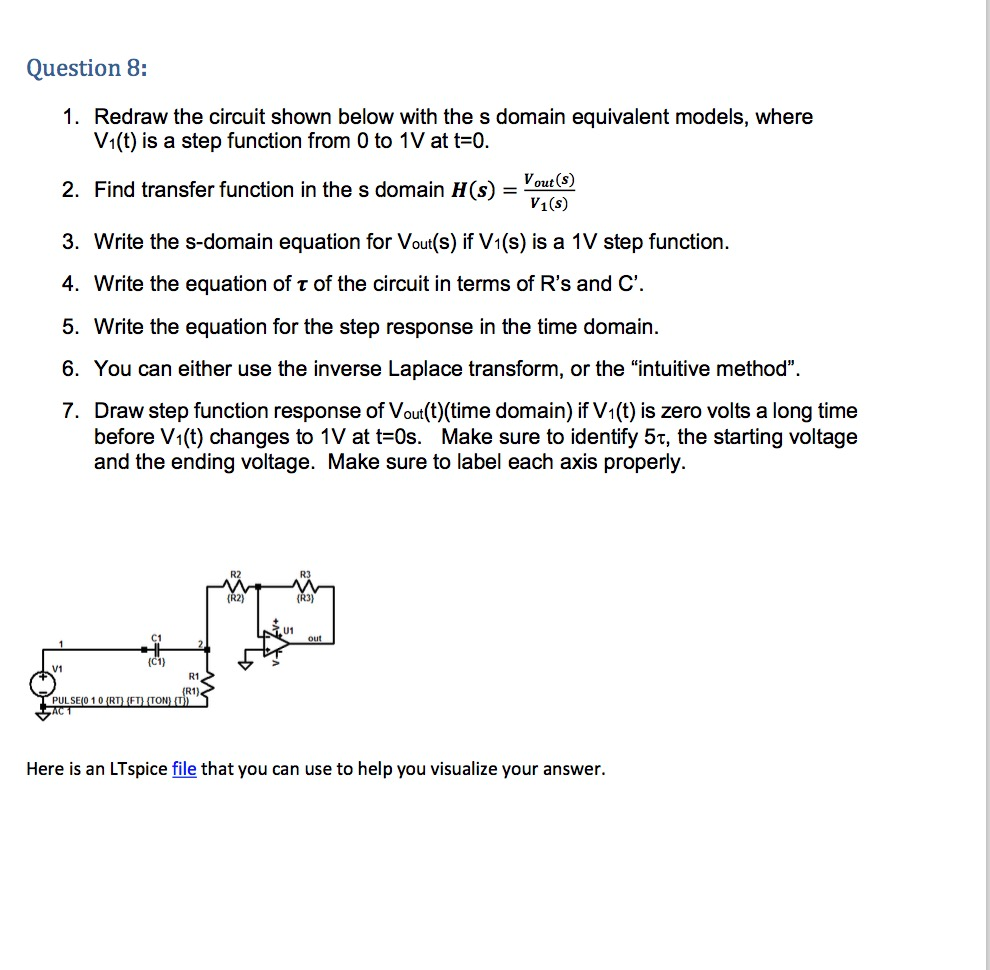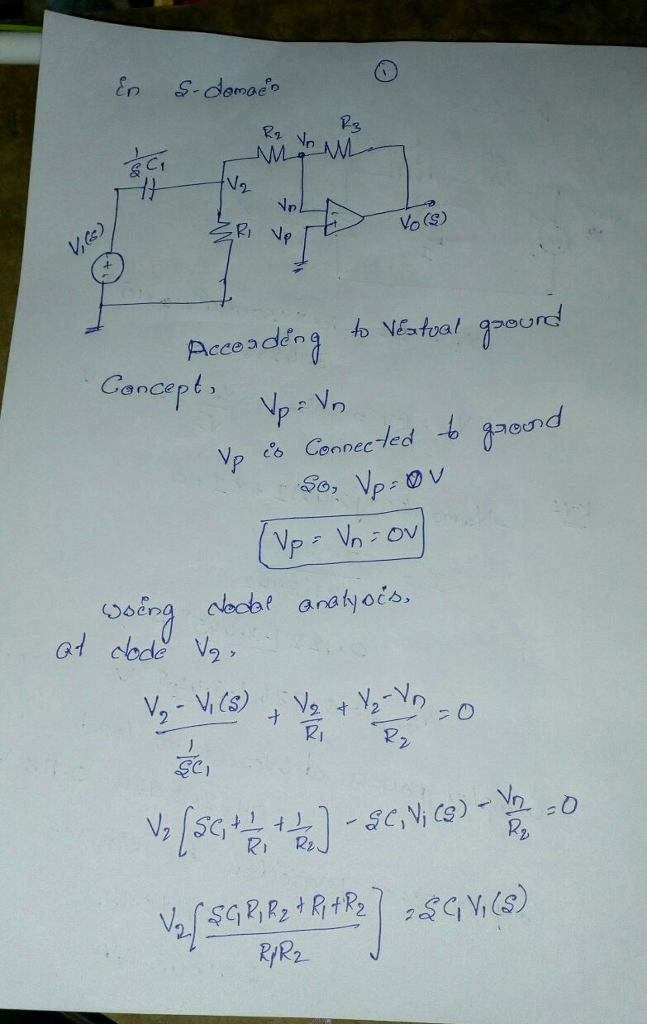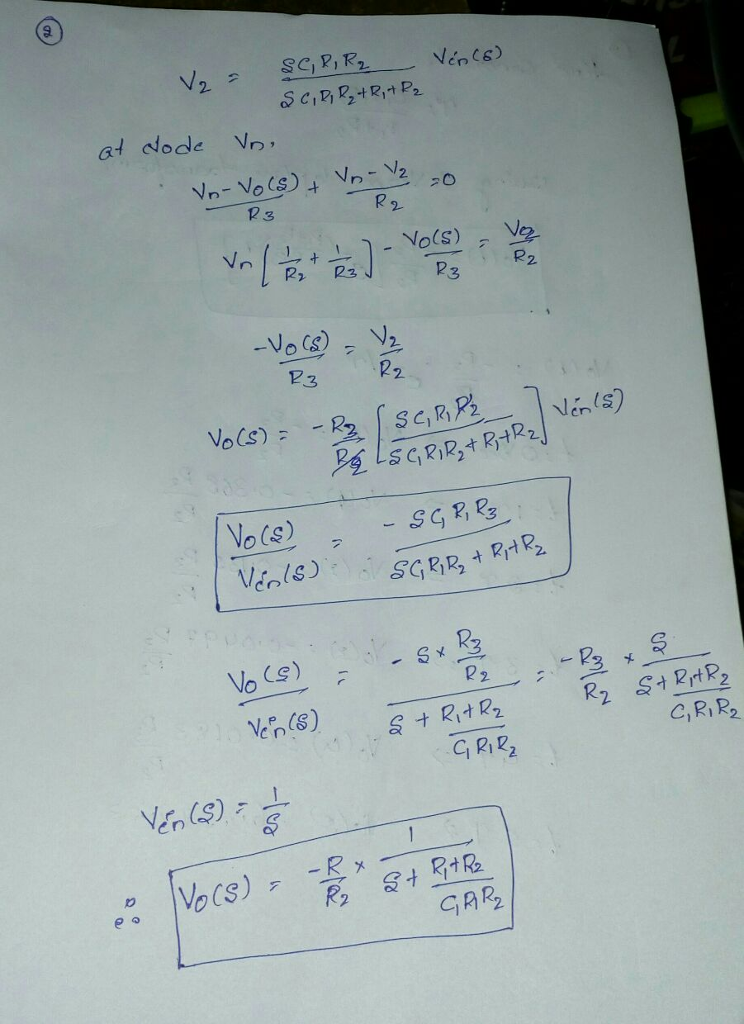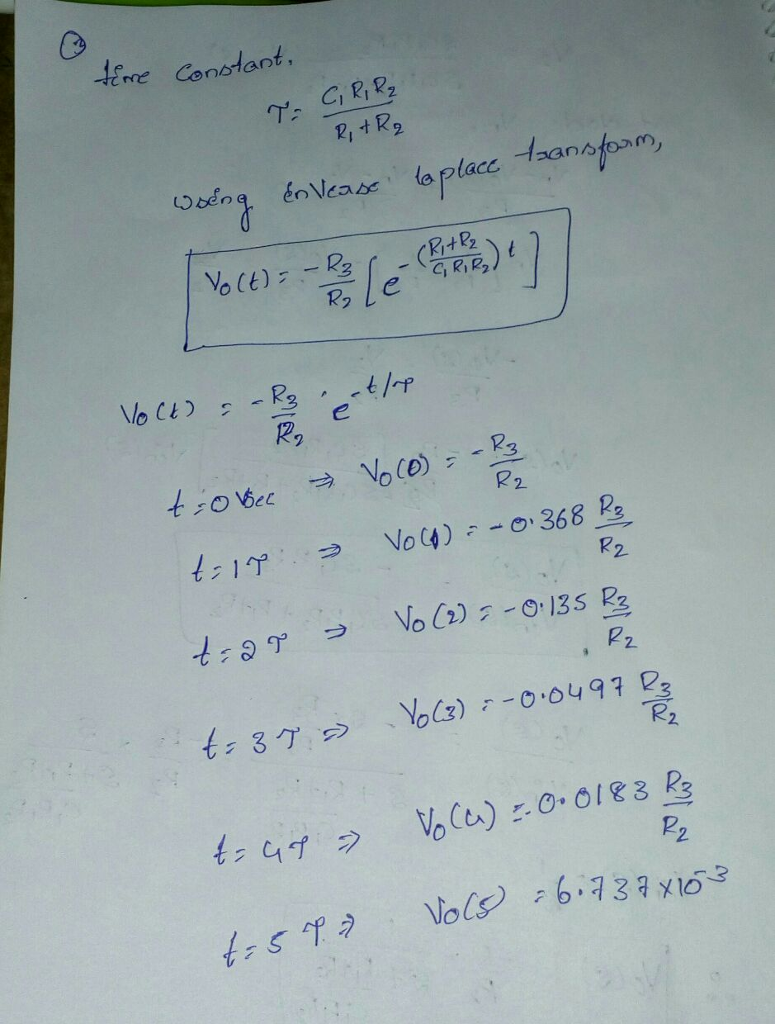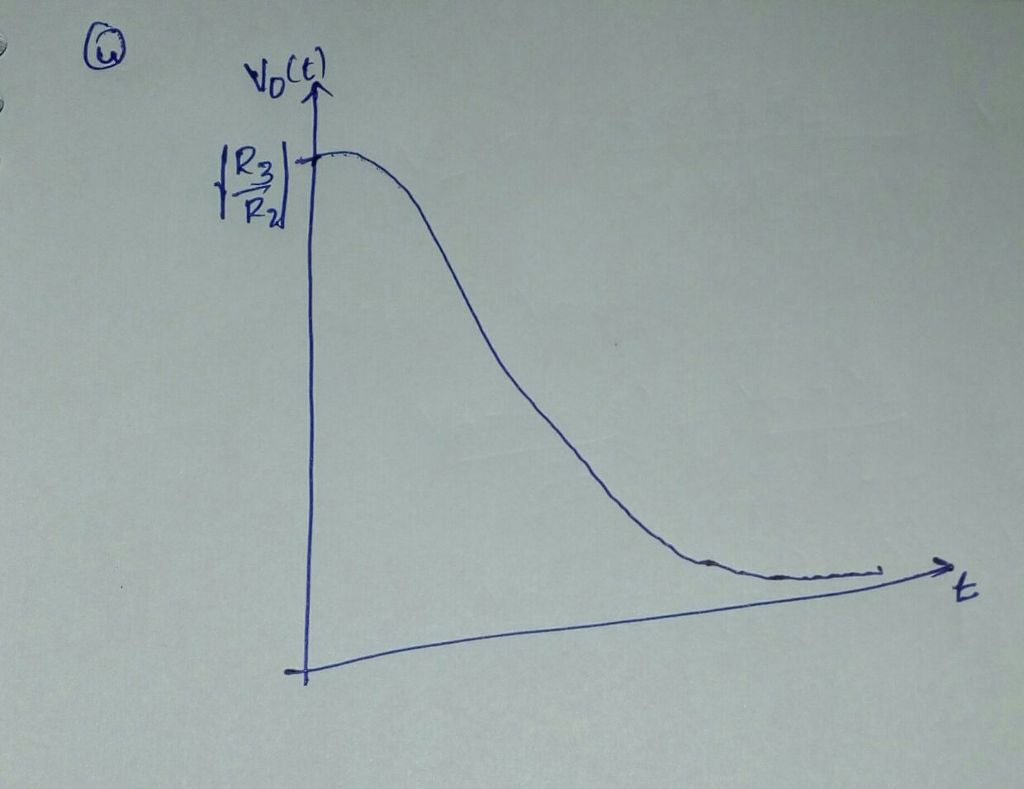#### Earn Coins

Coins can be redeemed for fabulous gifts.

Similar Homework Help Questions
• ### Q11 (20pts.): For the circuit shown below Derive the transfer function, H(s) assuming the OPAMP's transfer...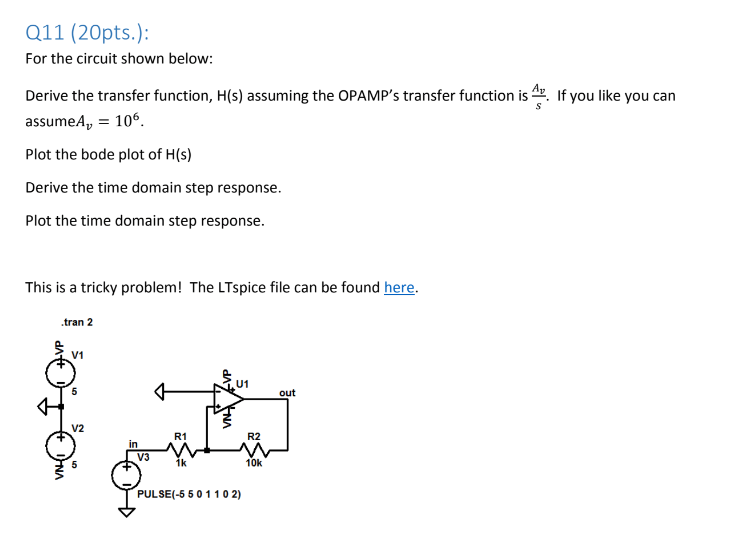Q11 (20pts.): For the circuit shown below Derive the transfer function, H(s) assuming the OPAMP's transfer function is. If you like you can assumeA 106 Plot the bode plot of H(s) Derive the time domain step response. Plot the time domain step response This is a tricky problem! The LTspice file can be found here. .tran 2 V1 U1 out V2 R1 R2 in V3 1k 10k PULSE(-5 50 1102)

• ### Problem 1: /25 For the circuit shown below, use frequency-domain circuit analysis techniques to determine (a)...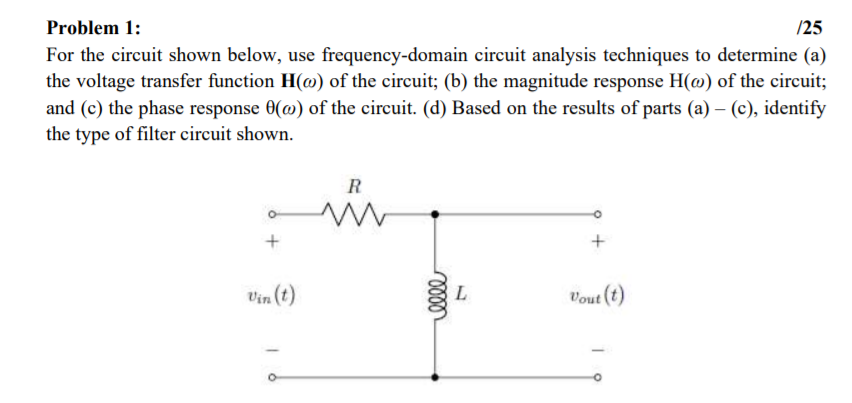Problem 1: /25 For the circuit shown below, use frequency-domain circuit analysis techniques to determine (a) the voltage transfer function Ho) of the circuit; (b) the magnitude response H(o) of the circuit; and (c) the phase response (0) of the circuit. (d) Based on the results of parts (a) - (c), identify the type of filter circuit shown. R + Vin(t) llll L Vout(t)

• ### Purpose: Use Laplace transforms to find the time domain response of a RLC band-pass filter to...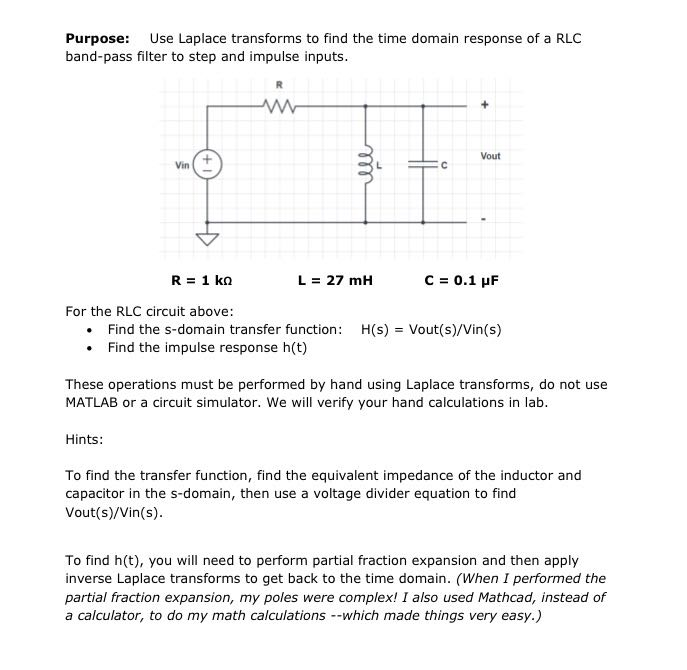Purpose: Use Laplace transforms to find the time domain response of a RLC band-pass filter to step and impulse inputs Vout Vin L=27 mH For the RLC circuit above Find the s-domain transfer function: Find the impulse response h(t) H(s) = Vout(s)/Vin(s) · These operations must be performed by hand using Laplace transforms, do not use MATLAB or a circuit simulator. We will verify your hand calculations in lab. Hints: To find the transfer function, find the equivalent impedance of...

• ### Problem 3: /25 For the circuit shown below, use frequency-domain circuit analysis techniques to determine (a)...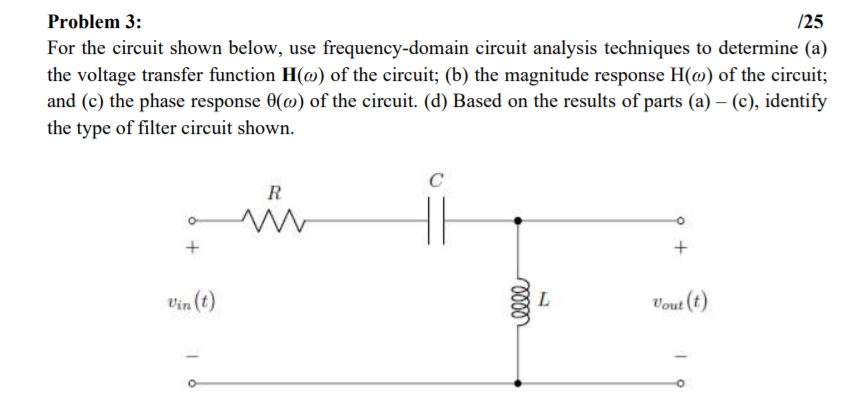Problem 3: /25 For the circuit shown below, use frequency-domain circuit analysis techniques to determine (a) the voltage transfer function Ho) of the circuit; (b) the magnitude response H(@) of the circuit; and (c) the phase response (0) of the circuit. (d) Based on the results of parts (a) - (c), identify the type of filter circuit shown. с R + + Vin(t) 0000 L Vout(t)

• ### For the circuit shown below: In terms of Vo, R1, R2, R3, R4, C1, t, and s what is the time domain...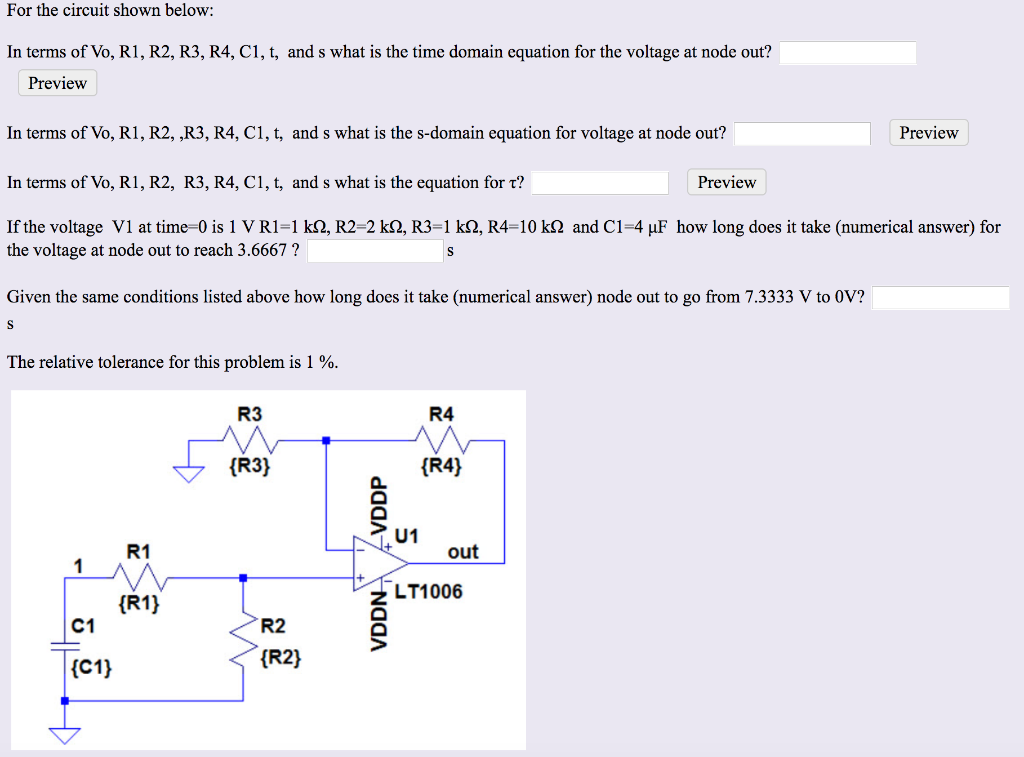For the circuit shown below: In terms of Vo, R1, R2, R3, R4, C1, t, and s what is the time domain equation for the voltage at node out? Preview In terms of Vo, R1, R2, ,R3, R4, C1, t, and s what is the s-domain equation for voltage at node out? In terms of Vo, RI, R2, R3, R4, CI, t, and s what is the equation for τ? If the voltage V1 at time-0 is 1 V R1-1...

• ### Problem 4: /25 For the circuit shown below, use frequency-domain circuit analysis techniques to determine (a)...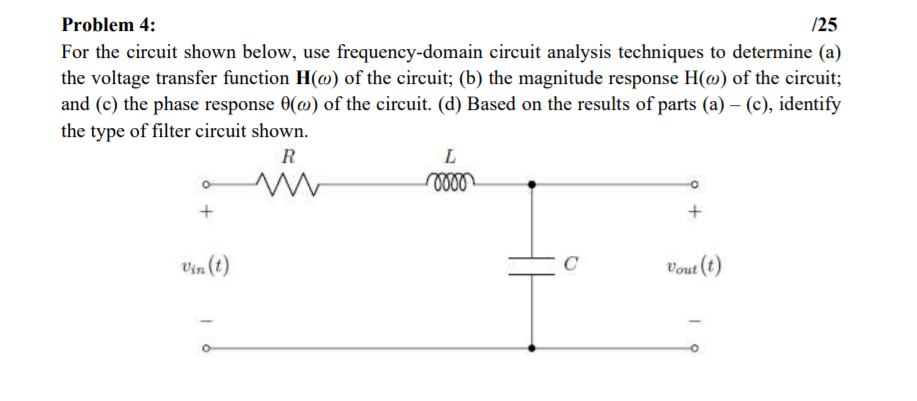Problem 4: /25 For the circuit shown below, use frequency-domain circuit analysis techniques to determine (a) the voltage transfer function Hw) of the circuit; (b) the magnitude response H(o) of the circuit; and (c) the phase response (0) of the circuit. (d) Based on the results of parts (a) - (c), identify the type of filter circuit shown. R L 10000 + + Vout(t)

• ### Problem 2: /25 For the circuit shown below, use frequency-domain circuit analysis techniques to determine (a)...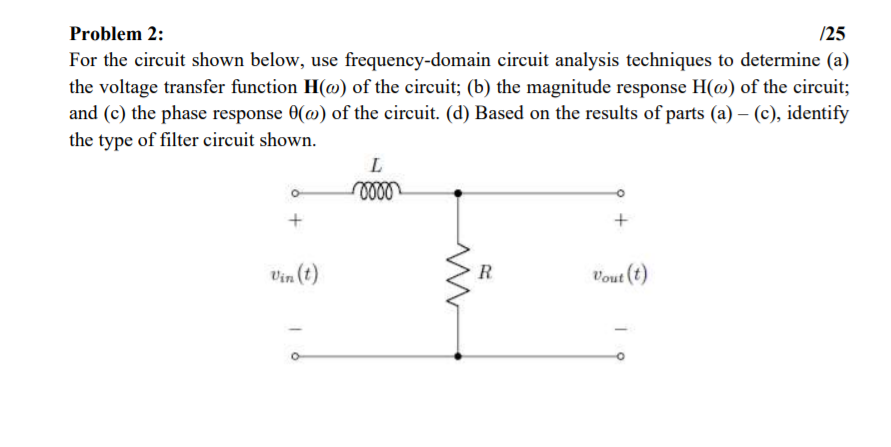Problem 2: /25 For the circuit shown below, use frequency-domain circuit analysis techniques to determine (a) the voltage transfer function H(o) of the circuit; (b) the magnitude response H(o) of the circuit; and (c) the phase response (0) of the circuit. (d) Based on the results of parts (a) - (c), identify the type of filter circuit shown. L 10000 + + R Vout(t)

• ### For the circuit shown below: Preview In terms of Vo, R1, R2, R3, R4 C1, t, and s what is the time domain equation for the voltage at node out? Preview and s what is the s-domain equation the voltage...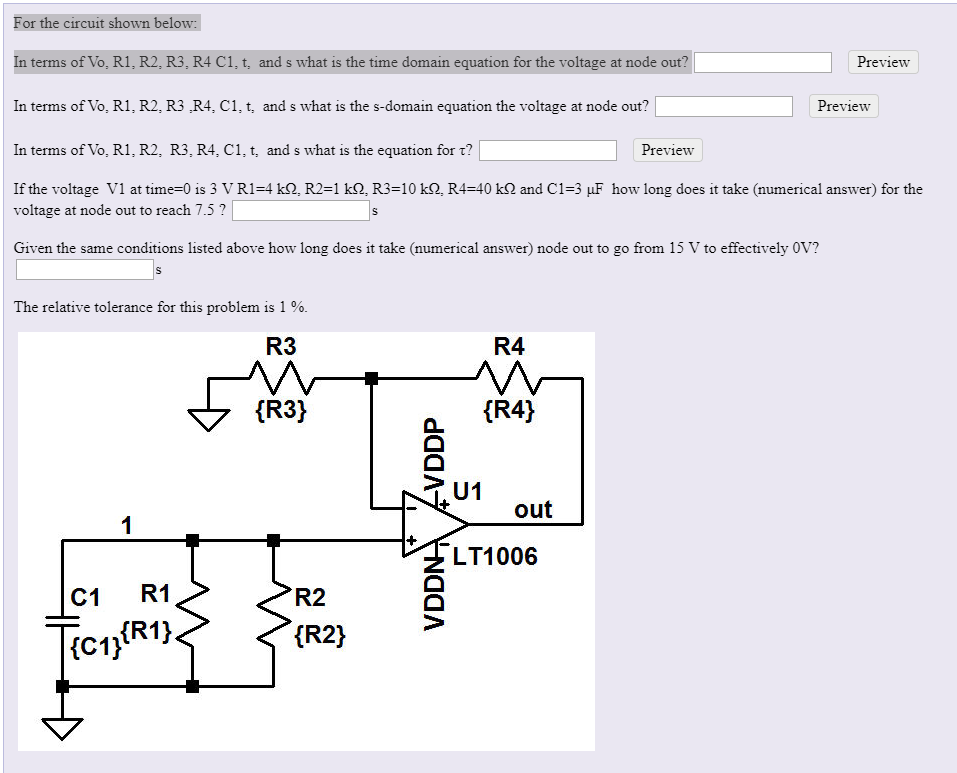For the circuit shown below: Preview In terms of Vo, R1, R2, R3, R4 C1, t, and s what is the time domain equation for the voltage at node out? Preview and s what is the s-domain equation the voltage at node out? In terms of Vo, RI, R2, R3,R4, C1, t, Preview and s what is the equation for τ? In terms ofVo, RI, R2, R3, R4, Cl, t, Ifthe voltage V1 at time-0 is 3 V RI=4 kQ,...

• ### Q1) Find the equivalent impedance of the circuit below in the S-domain. UC2F 30.5 H is(t)...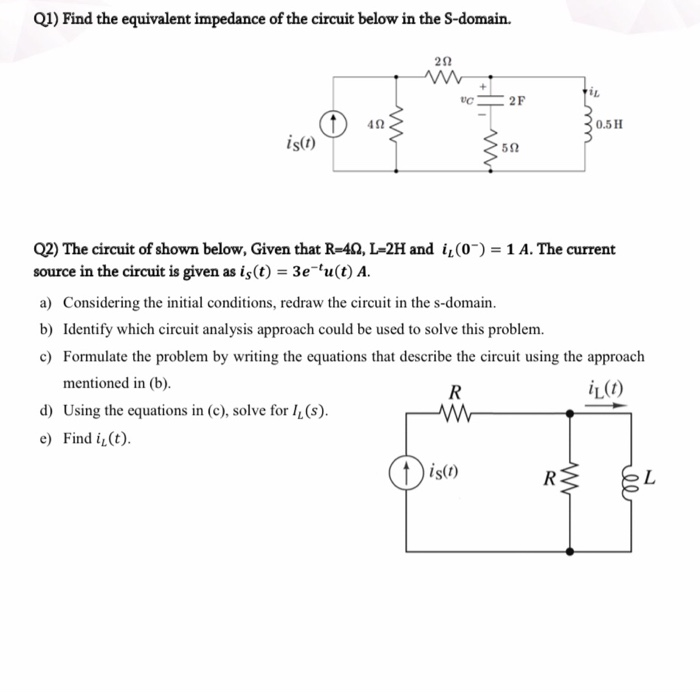Q1) Find the equivalent impedance of the circuit below in the S-domain. UC2F 30.5 H is(t) Q2) The circuit of shown below, Given that R-422, L=2H and i (0-) = 1 A. The current source in the circuit is given as is(t) = 3e 'u(t) A. a) Considering the initial conditions, redraw the circuit in the s-domain. b) Identify which circuit analysis approach could be used to solve this problem. c) Formulate the problem by writing the equations that describe...

• ### 1. Consider the circuit shown below. Cl e, (0) c, e。(t) Find the transfer function below using t...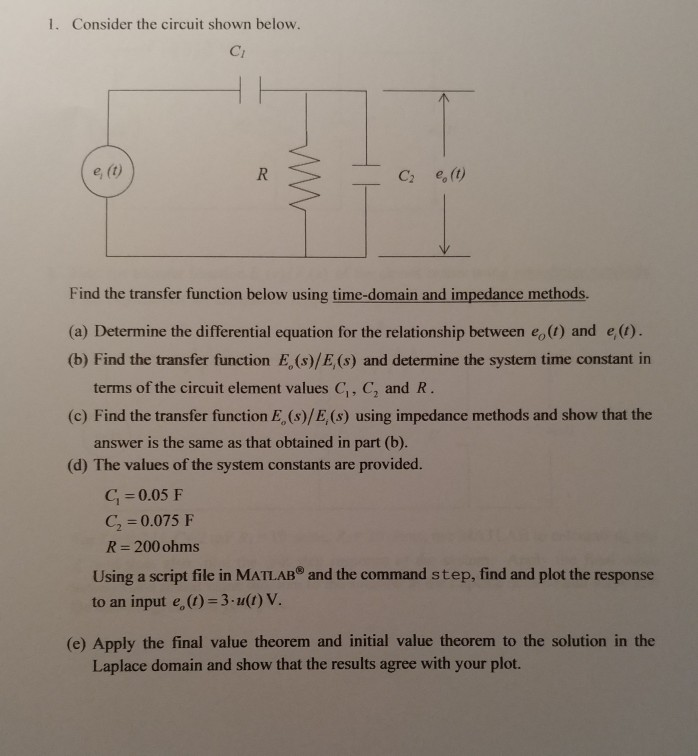Please help with this dynamics circuit analysis. Please show work and explain. Thank you!! 1. Consider the circuit shown below. Cl e, (0) c, e。(t) Find the transfer function below using time-domain and impedance methods. (a) Determine the differential equation for the relationship between eo(1) and e(1) (b) Find the transfer function E, (s)/E,(s) and determine the system time constant in terms of the circuit element values C, C, and R 17 2 (c) Find the transfer function E, (s)/E,...